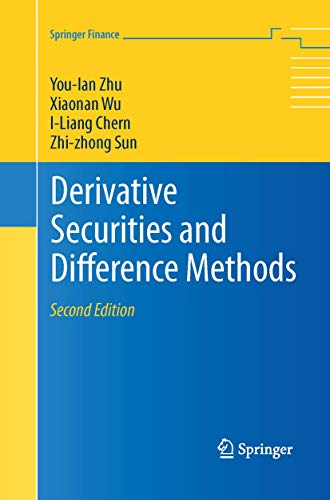• Free Shipping on all orders in Australia
• Over 7 million books in stock
• Proud to be B-Corp
• We aim to be carbon neutral by 2022
• Over 120,000 Trustpilot reviews
Item 1 of 0# Derivative Securities and Difference Methods by You-lan Zhu

Condition - New
218,29 \$
Only 2 left

## Summary

This book is mainly devoted to finite difference numerical methods for solving partial differential equations (PDEs) models of pricing a wide variety of financial derivative securities.

This book will be specially printed for your order, which means it may take a little longer to arrive (5 days). We do this to cut down on waste & to help protect our planet.

## Derivative Securities and Difference Methods Summary

### Derivative Securities and Difference Methods by You-lan Zhu

This book is mainly devoted to finite difference numerical methods for solving partial differential equations (PDEs) models of pricing a wide variety of financial derivative securities. With this objective, the book is divided into two main parts.

In the first part, after an introduction concerning the basics on derivative securities, the authors explain how to establish the adequate PDE boundary value problems for different sets of derivative products (vanilla and exotic options, and interest rate derivatives). For many option problems, the analytic solutions are also derived with details. The second part is devoted to explaining and analyzing the application of finite differences techniques to the financial models stated in the first part of the book. For this, the authors recall some basics on finite difference methods, initial boundary value problems, and (having in view financial products with early exercise feature) linear complementarity and free boundary problems. In each chapter, the techniques related to these mathematical and numerical subjects are applied to a wide variety of financial products. This is a textbook for graduate students following a mathematical finance program as well as a valuable reference for those researchers working in numerical methods in financial derivatives. For this new edition, the book has been updated throughout with many new problems added. More details about numerical methods for some options, for example, Asian options with discrete sampling, are provided and the proof of solution-uniqueness of derivative security problems and the complete stability analysis of numerical methods for two-dimensional problems are added.

Review of first edition:

"...the book is highly well designed and structured as a textbook for graduate students following a mathematical finance program, which includes Black-Scholes dynamic hedging methodology to price financial derivatives. Also, it is a very valuable reference for those researchers working in numerical methods in financial derivatives, either with a more financial or mathematical background." -- MATHEMATICAL REVIEWS

## Derivative Securities and Difference Methods Reviews

"It is mainly devoted to finite difference methods, and it is intended for researchers as well as graduate students. It is the most complete and useful book on the subject I have seen. ... Derivative Securities and Difference Methods is a really good book that anyone studying or working in this field should own." (Anita Mayo, SIAM Review, Vol. 57 (1), March, 2015)

"This book is devoted to pricing financial derivatives with a partial differential equation approach. It has two parts, each with four chapters. ... The book covers a variety of topics in finance, such as forward and futures contracts, the Black-Scholes model, European and American type options, free boundary problems, barrier options, lookback options, multi-asset options, interest rate models, interest rate derivatives, swaps, swaptions, caps, floors, and collars. The treatment is mathematically rigorous. There are exercises at the end of each chapter." (Elias Shiu, Zentralblatt MATH, Vol. 1061 (12), 2005)

"This book is mainly devoted to finite difference numerical methods for solving partial differential equations (PDEs) models of pricing a wide variety of financial derivative securities... the book is highly well designed and structured as a textbook for graduate students following a mathematical finance program, which includes Black-Scholes dynamic hedging methodology to price financial derivatives. Also, it is a very valuable reference for those researchers working in numerical methods in financial derivatives, either with a more financial or mathematical background." -- MATHEMATICAL REVIEWS

You-Lan Zhu is a Professor of Mathematics at the University of North Carolina at Charlotte. Xiaonan Wu is a Professor of Mathematics at Hong Kong Baptist University. I-Liang Chern is a Professor of Mathematics at National Taiwan University. Zhi-zhong Sun is a Professor of Mathematics at Southeast University.

Introduction.- European Style Derivatives.- American Style Derivatives.- Exotic Options.- Interest Rate Derivative Securities.- Basic Numerical Methods.- Finite Difference Methods.- Initial-Boundary Value and LC Problems.- Free-Boundary Problems.- Interest Rate Modeling.

NLS9781489990938
9781489990938
1489990933
Derivative Securities and Difference Methods by You-lan Zhu
New
Paperback
Springer-Verlag New York Inc.
2015-08-05
647
N/A
Book picture is for illustrative purposes only, actual binding, cover or edition may vary.
This is a new book - be the first to read this copy. With untouched pages and a perfect binding, your brand new copy is ready to be opened for the first time